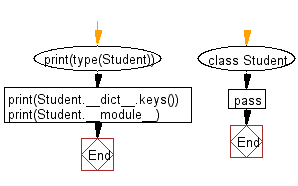﻿ Python: Simple Python class named Student and display its type - w3resource# Python: Simple Python class named Student and display its type

## Python Class ( Basic ): Exercise-7 with Solution

Write a simple Python class named Student and display its type. Also, display the __dict__ attribute keys and the value of the __module__ attribute of the Student class.
The pass statement does nothing. It can be used when a statement is required syntactically but the program requires no action. 'pass' can be used is as a place-holder for a function or conditional body when you are working on new code, allowing you to keep thinking at a more abstract level.

Sample Solution:

Python Code:

``````class Student:
pass
print(type(Student))
print(Student.__dict__.keys())
print(Student.__module__)
```
```

Sample Output:

```<class 'type'>
dict_keys(['__module__', '__dict__', '__weakref__', '__doc__'])
__main__
```

Flowchart:## Visualize Python code execution:

The following tool visualize what the computer is doing step-by-step as it executes the said program:

Python Code Editor:

What is the difficulty level of this exercise?

Test your Programming skills with w3resource's quiz.

﻿

## Python: Tips of the Day

The Zip() Function:

```>>> students = ('John', 'Mary', 'Mike')
>>> ages = (15, 17, 16)
>>> scores = (90, 88, 82, 17, 14)
>>> for student, age, score in zip(students, ages, scores):
...     print(f'{student}, age: {age}, score: {score}')
...
John, age: 15, score: 90
Mary, age: 17, score: 88
Mike, age: 16, score: 82
>>> zipped = zip(students, ages, scores)
>>> a, b, c = zip(*zipped)
>>> print(b)
(15, 17, 16)
```